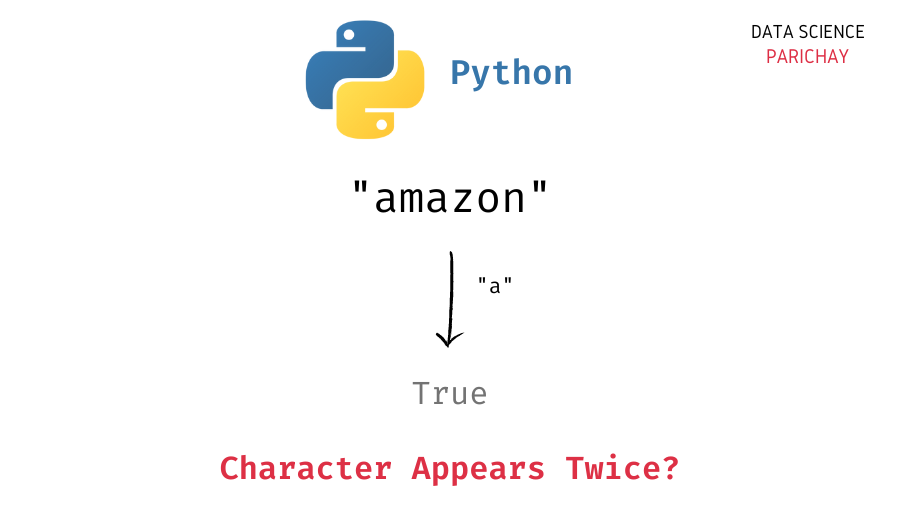# Python – Check If a Character Appears Twice in String

In this tutorial, we will look at how to check whether a character appears twice in a string or not in Python with the help of some examples.

## How to check if a character appears twice in a string?

Depending upon your use case, you might be checking for one of the following two things –

• Whether the character appears exactly twice in the string.
• Whether the character appears at least twice in the string.

To solve for both the above scenarios, you need to count the frequency of the given character in the string. To count the frequency of a character in a string, you can –

1. Iterate through the string and maintain a count of the number of times you find the given character.
2. Use `Counter` from the `collections` module in Python to get the count of each character in the string.

## Examples

Let’s now look at some examples of using the above methods. First, we will create a few strings that we will be using throughout this tutorial.

```# create strings
s1 = "apple"
s2 = "amazon"

# display the strings
print(s1)
print(s2)
print(s3)```

Output:

```apple
amazon

Here, we created three strings – `s1`, `s2`, and `s3`. Notice that the character `'a'` is present once in the string `s1`, twice in the string `s2`, and thrice in the string `s3`.

Note that the methods mentioned below are case-sensitive, so, if you want to count the frequency of a character in a case-insensitive manner, convert the strings to lowercase before proceeding.

### Example 1 – Check if a character appears exactly twice in a string

Here, we want to check if the character under consideration occurs exactly two times (no more, no less) in the string.

📚 Data Science Programs By Skill Level

Introductory

Intermediate ⭐⭐⭐

🔎 Find Data Science Programs 👨‍💻 111,889 already enrolled

Disclaimer: Data Science Parichay is reader supported. When you purchase a course through a link on this site, we may earn a small commission at no additional cost to you. Earned commissions help support this website and its team of writers.

Now, there are a few ways to count the frequency of a character in a string. Let’s look at two such methods.

In the first method, we iterate through the string and keep a count of the occurrence of the given character.

```# function to count frequecy of ch in s
def get_char_frequecy(ch, s):
count = 0
for c in s:
if c == ch:
count += 1
return count

# check if 'a' occurs exactly twice
print(get_char_frequecy('a', s1) == 2)
print(get_char_frequecy('a', s2) == 2)
print(get_char_frequecy('a', s3) == 2)```

Output:

```False
True
False```

Here, we created a function to count the frequency of a given character in a given string and then check if the resulting frequency is exactly equal to `2` or not.

We get `False` for `s1` and `s3` and `True` for `s2` (as `s2` contains `'a'` exactly) twice.

Alternatively, you can use `Counter` from the `collections` module in Python to get the count of each character in the string.

```from collections import Counter

# get frequecy map of each character
c1 = Counter(s1)
c2 = Counter(s2)
c3 = Counter(s3)

# check if 'a' occurs exactly twice
print(c1['a'] == 2)
print(c2['a'] == 2)
print(c3['a'] == 2)```

Output:

```False
True
False```

We create a `Counter` object which you can think of as a dictionary mapping each unique character in the string to its respective frequency. We then check if the count of `'a'` is exactly `2` or not.

We get the same result as above.

### Example 2 – Check if a character appears at least twice in a string

Here, we want to check whether the character appears two or more times in the string or not.

Much of the logic remains the same, we first determine the count of the character in the string and then check if the count is greater than or equal to `2` or not.

We use the same methods as in the above example to get the count of a character.

First, using a `for` loop.

```# check if 'a' occurs at least twice
print(get_char_frequecy('a', s1) >= 2)
print(get_char_frequecy('a', s2) >= 2)
print(get_char_frequecy('a', s3) >= 2)```

Output:

```False
True
True```

We get `False` for `s1` and `True` for `s2` and `s3` (as `'a'` occurs at least twice in them).

Alternatively, we can use `Counter` to get the counts.

```# check if 'a' occurs at least twice
print(c1['a'] >= 2)
print(c2['a'] >= 2)
print(c3['a'] >= 2)```

Output:

```False
True
True```

We get the same result as above.

You might also be interested in –

•